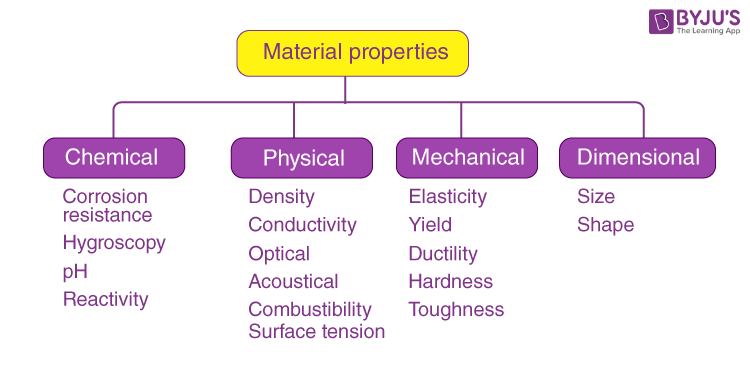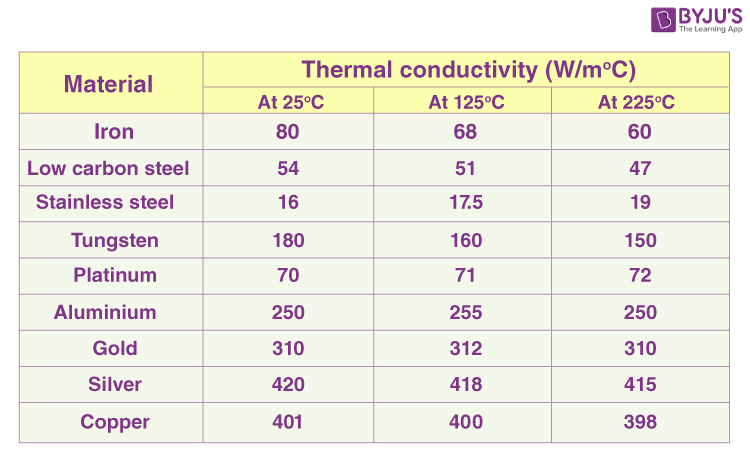# Thermal Properties of Materials

The matter has properties or characteristics by which they can be identified. The major properties of materials can be categorized under:

• Mechanical properties of materials
• Chemical properties of materials
• Physical properties of materials
• Dimensional properties of materials
• Thermal properties of materials## What are Thermal Properties of Materials

Thermal properties are those properties of a material which is related to its conductivity of heat. In other words, these are the properties that are exhibited by a material when the heat is passed through it. Thermal properties come under the broader topic of the physical properties of materials.

Thermal properties of material decide how it reacts when it is subjected to heat fluctuation (excessive heat or very low heat, for example). The major components of thermal properties are:

• Heat capacity
• Thermal Expansion
• Thermal conductivity
• Thermal stress

## What is Heat Capacity?

The heat capacity of a material can be defined as the amount of heat required to change the temperature of the material by one degree. The amount of heat is generally expressed in joules or calories and the temperature in Celsius or Kelvin.

In order to calculate the heat capacity of materials with a given dimension, Molar heat capacity or Specific heat capacity is used.

### Heat Capacity Formula

 Q = m c ΔT

Where,

• Q is the heat capacity in J
• m is the mass in g
• c is the specific heat in J.K-1
• ΔT is the temperature change in °K

## Major Components of Thermal Properties

### Thermal Expansion

When heat is passed through a material, its shape changes. Generally, a material expands when heated. This property of a material is called thermal expansion. There can be a change in the area, volume, and shape of the material. For example, railway tracks often expand and as a result, get misshapen due to extreme heat.Effect of thermal expansion on a railway track

### Thermal conductivity

It is the property of a material to conduct heat through itself. Materials with high thermal conductivity will conduct more heat than the ones with low conductivity. For example, an iron rod will conduct more heat than normal window glass.Some materials do not conduct heat at all because of the insulating properties of materials.

### Thermal stress

The stress experienced by a body due to either thermal expansion or contraction is called thermal stress. It can be potentially destructive in nature as it can make the material explode. For example, cracks can be seen on roads where the heat is extreme. The crack is a result of thermal stress.

## Frequently Asked Questions – FAQs

Q1

### How are properties of materials classified?

Properties of materials are classified into:
• Mechanical properties of materials
• Chemical properties of materials
• Physical properties of materials
• Dimensional properties of materials
• Thermal properties of materials
• Q2

### What are the major components of thermal properties?

Major components of thermal properties are:
• Heat capacity
• Thermal Expansion
• Thermal conductivity
• Thermal stress
• Q3

### What is the formula to calculate the heat capacity?

The formula gives heat capacity: Q = m c ΔT
Where,
Q – heat capacity
m – mass
c – specific heat
ΔT – temperature change
Q4

### State true or false: Materials with high thermal conductivity conducts less heat.

False. Materials with high thermal conductivity will conduct more heat.
Q5

### What is thermal stress?

The stress experienced by a body due to contraction or thermal expansion is known as thermal stress.